NEET  >  Previous Year Questions: Centre of Mass

# Previous Year Questions: Centre of Mass

Test Description

## 11 Questions MCQ Test Physics Class 11 | Previous Year Questions: Centre of Mass

Previous Year Questions: Centre of Mass for NEET 2022 is part of Physics Class 11 preparation. The Previous Year Questions: Centre of Mass questions and answers have been prepared according to the NEET exam syllabus.The Previous Year Questions: Centre of Mass MCQs are made for NEET 2022 Exam. Find important definitions, questions, notes, meanings, examples, exercises, MCQs and online tests for Previous Year Questions: Centre of Mass below.
Solutions of Previous Year Questions: Centre of Mass questions in English are available as part of our Physics Class 11 for NEET & Previous Year Questions: Centre of Mass solutions in Hindi for Physics Class 11 course. Download more important topics, notes, lectures and mock test series for NEET Exam by signing up for free. Attempt Previous Year Questions: Centre of Mass | 11 questions in 11 minutes | Mock test for NEET preparation | Free important questions MCQ to study Physics Class 11 for NEET Exam | Download free PDF with solutions
 1 Crore+ students have signed up on EduRev. Have you?
Previous Year Questions: Centre of Mass - Question 1

### Two block of masses 10 kg and 4 kg are connected by a spring of negligible mass and placed on a frictionless horizontal surface. An impulse gives a velocity of 14 m/s to the heavier block in the direction of the lighter block. The velocity of the centre of mass is    [IIT(Scr.)-2002]

Detailed Solution for Previous Year Questions: Centre of Mass - Question 1

Velocity of heavier block after the impulse = 14m/s
Velocity of lighter block after the impulse = 0 m/s
Thus velocity of COM = 14 x 10 + 0 x 4  / 10 + 4 = 10m/s

Previous Year Questions: Centre of Mass - Question 2

### STATEMENT-1 In an elastic collision between two bodies, the relative speed of the bodies after collision is equal to the relative speed before the collision. because STATEMENT-2 In an elastic collision, the linear momentum of the system is conserved

Previous Year Questions: Centre of Mass - Question 3

### The balls, having linear momenta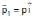and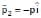, undergo a collision in free space. There is no external force acting on the balls. Let and be their final momenta. The following options(s) is (are) NOT allowed for any non-zero value of p, a1, a2, b1, b2, c1 and c2    [JEE 2008]

Detailed Solution for Previous Year Questions: Centre of Mass - Question 3

As given
P=P Î
P2​​=−PÎ
∴  Net Linear Momentum first before collision
Pi=P1​​+P2​​=PÎ−PÎ=0
∵  No external force is acting on the balls, momentum will remain conserved.
Pf′​=P1​​+P2​​=0
∴ In option (A)=P1​​+P2​​=(a1​+a2​)Î+(b1​+b2​)Î+c1​k=/0
Qc1​ in non-zero
∴  P1​​+P2​​=(a1​+a2​)Î +2b1​Î =0
Q b1​ is non zero

Previous Year Questions: Centre of Mass - Question 4

A small block of mass M moves on a frictionless surface of an inclined plane, as shown in figure. The angle of the incline suddenly changes from 60° to 30° at point B. The block is initially at rest at A. Assume that collisions between the block and the incline are totally inelastic (g = 10 m/s2). Figure :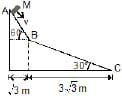The speed of the block at point B immediately after it strikes the second incline is

[JEE 2008]

Detailed Solution for Previous Year Questions: Centre of Mass - Question 4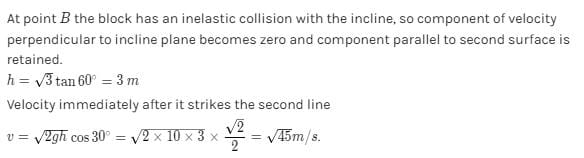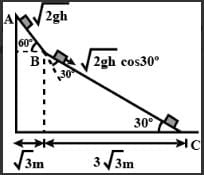Previous Year Questions: Centre of Mass - Question 5

A small block of mass M moves on a frictionless surface of an inclined plane, as shown in figure. The angle of the incline suddenly changes from 60° to 30° at point B. The block is initially at rest at A. Assume that collisions between the block and the incline are totally inelastic (g = 10 m/s2). Figure :The speed of the block at point C, immediately before it leaves the second incline is

[JEE 2008]

Detailed Solution for Previous Year Questions: Centre of Mass - Question 5

At point B since the block undergoes inelastic collision, the component of velocity perpendicular to the incline becomes zero and only the component of velocity parallel to the incline is left.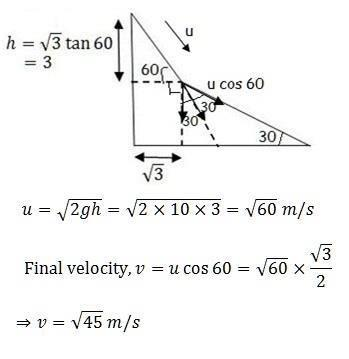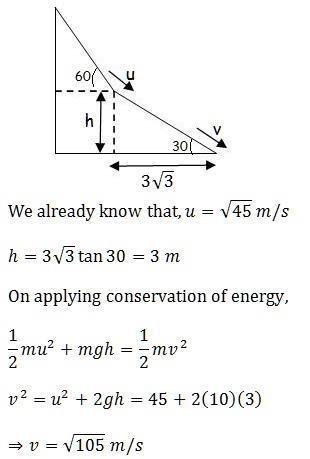Previous Year Questions: Centre of Mass - Question 6

A small block of mass M moves on a frictionless surface of an inclined plane, as shown in figure. The angle of the incline suddenly changes from 60° to 30° at point B. The block is initially at rest at A. Assume that collisions between the block and the incline are totally inelastic (g = 10 m/s2). Figure :

[JEE 2008]If collision between the block and the incline is completely elastic, then the vertical (upward) component of the velocity of the block at point B, immediately after it strikes the second incline is

Detailed Solution for Previous Year Questions: Centre of Mass - Question 6

v=√2g(3)=√60m/s
Along the plane velocity just after collision
vB=v cos 30°=√45m/s
vy= v sin 30° cos 30° − v cos 30° cos 60°
vy=0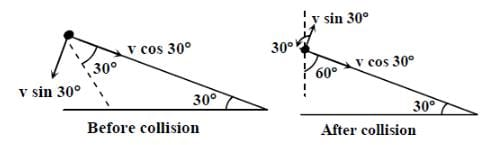Previous Year Questions: Centre of Mass - Question 7

Look at the drawing given in the figure which has been drawn with ink of uniform line-thickness. The mass of ink used to draw each of the two inner circles, and each of the two line segments is m. The mass of the ink unsed to draw the outer circle is 6m. The coordinates of the centres of the different parts are outer circle (0, 0), left inner circle (_a, a), right inner circle (a, a), vertical line (0, 0) and horizontal line (0, _ a). The y-coordinate of the centre of mass of the ink in this drawing is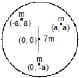[JEE 2009]

Detailed Solution for Previous Year Questions: Centre of Mass - Question 7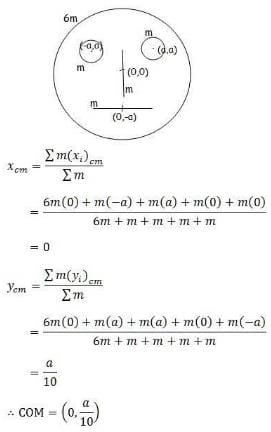Previous Year Questions: Centre of Mass - Question 8

Two small particles of equal masses start moving in opposite directions from a point A in a horizontal circle orbit. Their tangential velocities are v and 2v, respectively, as shown in the figure. Between collisions, the particles move with constant speeds. After making how many elastic collisions, other than that at A, these two particulars will again reach the point A ?[JEE 2009]

Detailed Solution for Previous Year Questions: Centre of Mass - Question 8

First collision will occur at an angle of 1200 from the starting point since one particle will cover angle twice of the other one and the sum of the two angles will be 3600. Next, due to elastic collision, velocities will exchange. Similarly, the next collision will occur at an angle 1200 from this position. Then the next collision will occur at A. Hence there will be 2 collisions before meeting again at A.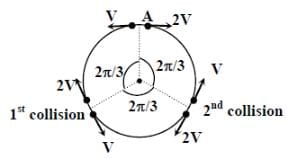Previous Year Questions: Centre of Mass - Question 9

Three objects Aand are kept in a straight line on a frictionless horizontal surface. These have masses m, 2and m, respectively. The object moves towardswith a speed 9 ms-1 and makess an elastic collision with it. There after, makes completely inelastic collision with C. All motions occur on the same straight line. Find the final speed (in ms-1 ) of the object C.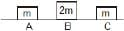[JEE 2009]

Detailed Solution for Previous Year Questions: Centre of Mass - Question 9

There is a quantity known as coefficient of restitution which can be defined as the ratio of relative velocity of separation (after collision) and relative velocity of approach (before collision). Mathematically it can be given as shown below. Also for a completely elastic collision, e = 1 and for a completely inelastic collision, e = 0.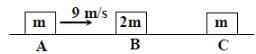After 1st collision
mvA​=mv′​A+2mv′B

−1=v′B​−v′A/0−vA ​​⇒v′B​=6m/s

After the 2nd collision
2mv′​B=(2m+m)VC​​⇒vC​=2​v’B/3​⇒vC​=4m/s

*Multiple options can be correct
Previous Year Questions: Centre of Mass - Question 10

A point mass of 1 kg collides elastically with a stationary point mass of 5 kg. After their collision, the 1 kg mass reverse its direction and moves with a speed of 2 ms-1. Which of the following statement(s) is (are ) correct for the system of these two masses ?

[JEE 2010]

Detailed Solution for Previous Year Questions: Centre of Mass - Question 10

By using the conservation of linear momentum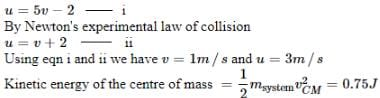Also, the total momentum is 3 kg m/s

*Multiple options can be correct
Previous Year Questions: Centre of Mass - Question 11

A ball of mass 0.2 kg rests on a vertical post of height 5 m. A bullet of mass 0.01 kg traveling with a velocity V m/s in a horizontal direction, hits the centre of the ball. After the collision, the ball and bullet travel independently. The ball hits the ground at a distance of 20 m and the bullet at a distance of 100 m from the foot of the post. The initial velocity V of the bullet is

[JEE 2011]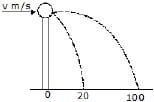## Physics Class 11

126 videos|449 docs|210 tests
 Use Code STAYHOME200 and get INR 200 additional OFF Use Coupon Code
Information about Previous Year Questions: Centre of Mass Page
In this test you can find the Exam questions for Previous Year Questions: Centre of Mass solved & explained in the simplest way possible. Besides giving Questions and answers for Previous Year Questions: Centre of Mass, EduRev gives you an ample number of Online tests for practice

## Physics Class 11

126 videos|449 docs|210 tests

### How to Prepare for NEET

Read our guide to prepare for NEET which is created by Toppers & the best Teachers